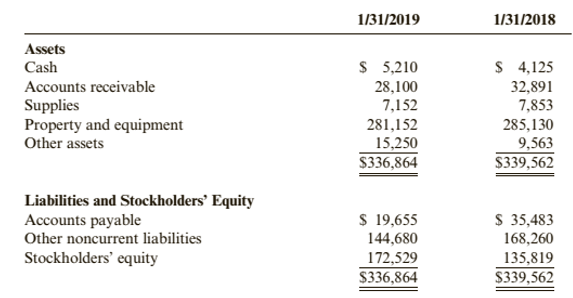Chapter 1, Problem 69.2C### Cornerstones of Financial Accounti...

4th Edition
Jay Rich + 1 other
ISBN: 9781337690881

#### Solutions

Chapter
Section### Cornerstones of Financial Accounti...

4th Edition
Jay Rich + 1 other
ISBN: 9781337690881
Textbook Problem
3 views

# Case 1-69 Financial Statement AnalysisAgency Rent-A-Car Inc. rents cars to customers whose vehicles are unavailable due to accident.theft, or repair (“Wheels while your car heals"). The company has a fleet of more than 40,000 cars located at 3'00 offices throughout the United States and Canada; Its balance sheets at January 31, 2019, and January 31, 2018. contain the following information (all dollar amounts are stated in thousands of dollars):Required:2. Assume that stockholders were paid dividends of $21,000 during 2019 and that there were no other changes in stockholders’ equity except for net income. How much net income did the business earn during the year? To determine Concept Introduction: Financial statements: Financial statements are prepared to summaries the account at the end of the period. The statements prepared are Income statement, Balance sheet, Statement of owner’s equity and Cash flows statements. Accounting equation: Accounting equation represents the mathematical relationship between assets, liabilities and equity. According to this equation, assets are equal to the sum of liabilities and equity. The formal for basic accounting equation is as follows: Assets = Liabilities + Equity Statement of Retained earnings: Items reported on a retained earnings statement are explained as follows: • Beginning Balance of Retained earnings is the ending balance of the retained earnings of the previous year. • Net Income or Net loss is the amount of net income earned or net loss incurred for the year. • Dividends Paid are taken for the year. • Ending Balance of Retained earnings is calculated as follows: Ending Balance of Retained earnings = Beginning Balance of Retained earnings + Net Income − Net income − Dividends Paid To Calculate: The Net income (loss) for the year 2019. Explanation The Net income (loss) for the year 2019 is calculated as follows:  Stockholder’s equity at the end of year 2019$ 172,529 Add: Dividends Paid during 2019

### Still sussing out bartleby?

Check out a sample textbook solution.

See a sample solution

#### The Solution to Your Study Problems

Bartleby provides explanations to thousands of textbook problems written by our experts, many with advanced degrees!

Get Started

#### What is structural independence, and why is it important?

Database Systems: Design, Implementation, & Management

#### Describe how a Wi-Fi network works.

Principles of Information Systems (MindTap Course List)

#### What do we mean by the transpose of a matrix?

Engineering Fundamentals: An Introduction to Engineering (MindTap Course List)

#### What is PPE?

Precision Machining Technology (MindTap Course List)